# Question 19 2 pts In the following ODE, y is the independent variable. dy der 5ytet...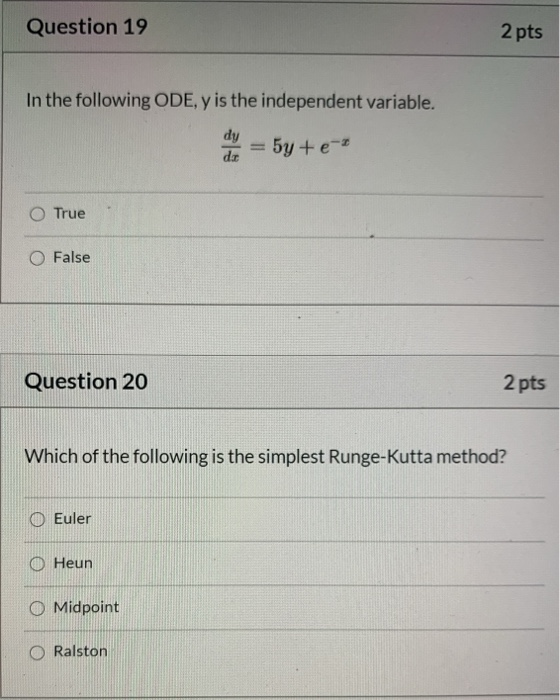Question 19 2 pts In the following ODE, y is the independent variable. dy der 5ytet True O False Question 20 2 pts Which of the following is the simplest Runge-Kutta method? Euler Heun O Midpoint Ralston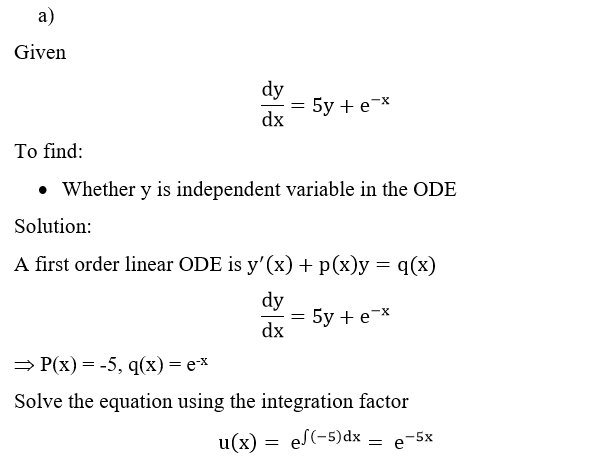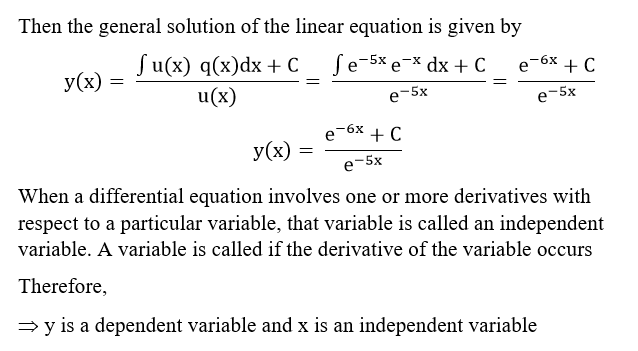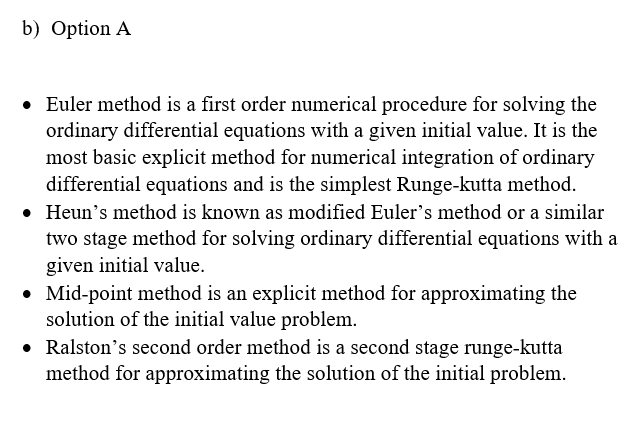##### Add Answer of: Question 19 2 pts In the following ODE, y is the independent variable. dy der 5ytet...
Similar Homework Help Questions
• ### Question 21 2 pts Which of the following is based on using the average of two...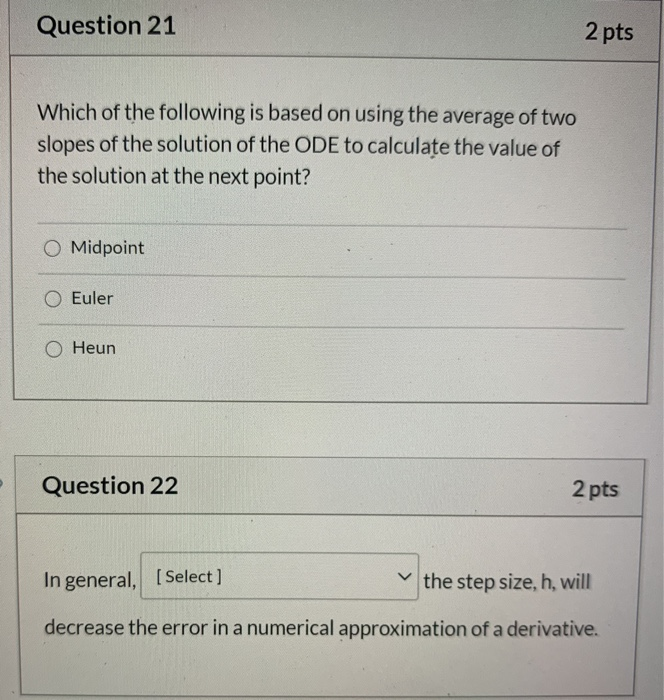Question 21 2 pts Which of the following is based on using the average of two slopes of the solution of the ODE to calculate the value of the solution at the next point? Midpoint O Euler O Heun Question 22 2 pts In general, [Select] the step size, h, will decrease the error in a numerical approximation of a derivative.

• ### Problem Thre: 125 points) Consider the following initial value problem: dy-2y+ t The y(0) -1 ea dt ical solution of the differential equation is: y(O)(2-2t+3e-2+1)y fr exoc the differential equat...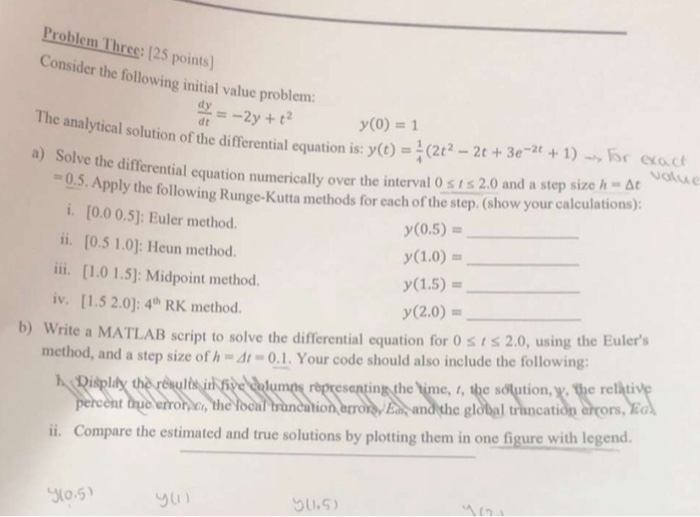Problem Thre: 125 points) Consider the following initial value problem: dy-2y+ t The y(0) -1 ea dt ical solution of the differential equation is: y(O)(2-2t+3e-2+1)y fr exoc the differential equation numerically over the interval 0 s i s 2.0 and a step size h At 0.5.A Apply the following Runge-Kutta methods for each of the step. (show your calculations) i. [0.0 0.5: Euler method ii. [0.5 1.0]: Heun method. ii. [1.0 1.5): Midpoint method. iv. [1.5 2.0): 4h RK method...

• ### (e) Consider the Runge-Kutta method in solving the following first order ODE: dy First, using Taylor series expansion, we have the following approximation of y evaluated at the time step n+1 as a...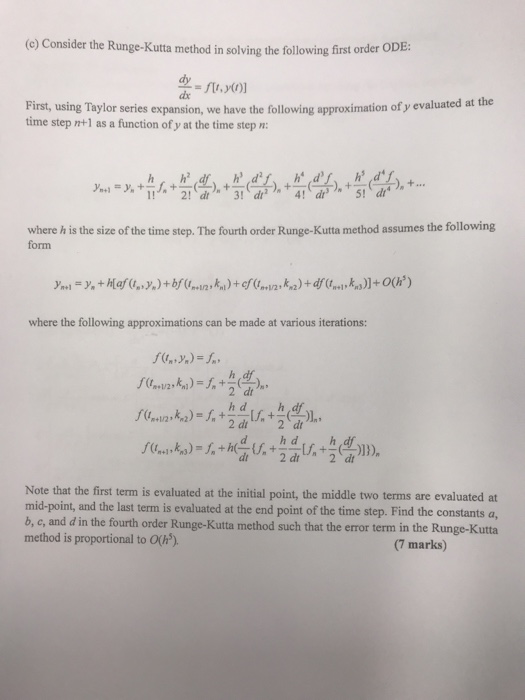(e) Consider the Runge-Kutta method in solving the following first order ODE: dy First, using Taylor series expansion, we have the following approximation of y evaluated at the time step n+1 as a function of y at the time step n: where h is the size of the time step. The fourth order Runge-Kutta method assumes the following form where the following approximations can be made at various iterations: )sh+รู้: ,f(t.ta, ),. Note that the first term is evaluated at...

• ### Consider the following Ordinary Differential Equation (ODE): dy = 0.3 * x2 + 0.04 * 26...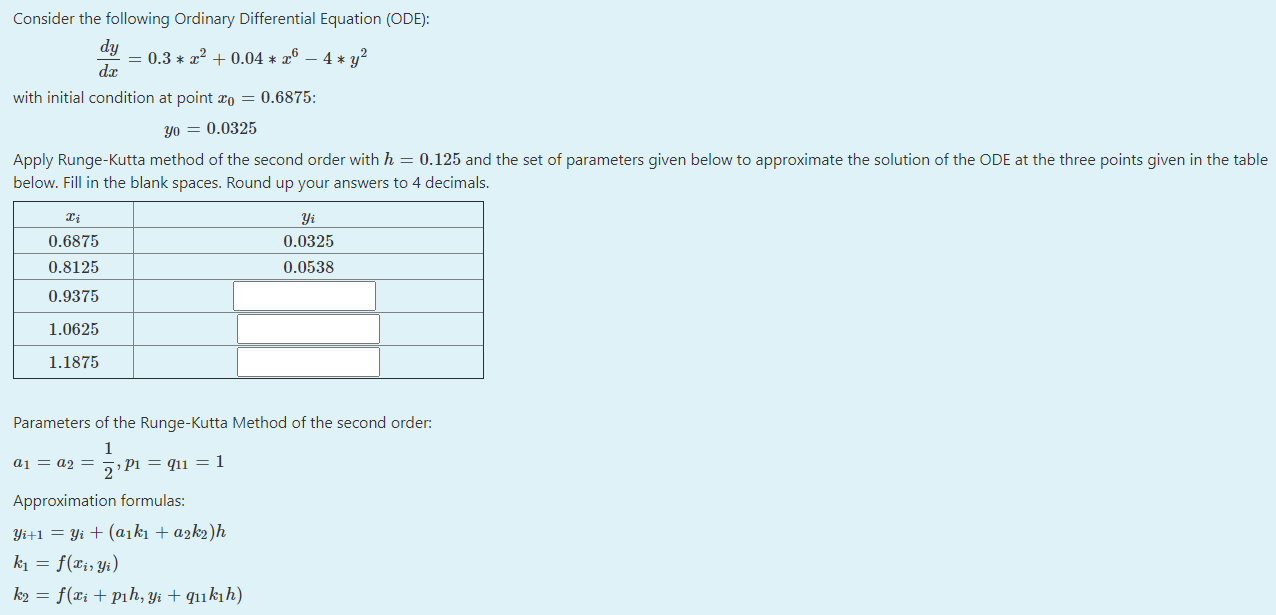Consider the following Ordinary Differential Equation (ODE): dy = 0.3 * x2 + 0.04 * 26 – 4* y? dx with initial condition at point 20 = 0.6875: yo = 0.0325 Apply Runge-Kutta method of the second order with h = 0.125 and the set of parameters given below to approximate the solution of the ODE at the three points given in the table below. Fill in the blank spaces. Round up your answers to 4 decimals. Yi 0.0325 0.6875...

• ### Numerical Methods Consider the following IVP dy=0.01(70-y)(50-y), with y(0)-0 (a) [10 marks Use the Runge-Kutta method of order four to obtain an approximate solution to the ODE at the points t-0.5 an...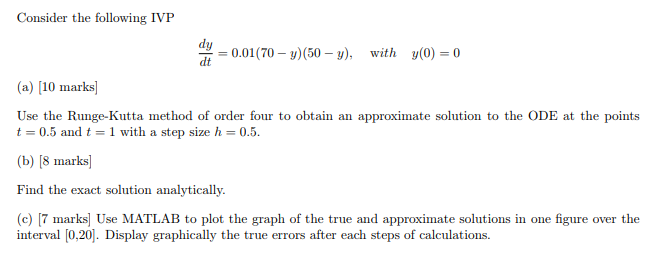Numerical Methods Consider the following IVP dy=0.01(70-y)(50-y), with y(0)-0 (a) [10 marks Use the Runge-Kutta method of order four to obtain an approximate solution to the ODE at the points t-0.5 and t1 with a step sizeh 0.5. b) [8 marks Find the exact solution analytically. (c) 7 marks] Use MATLAB to plot the graph of the true and approximate solutions in one figure over the interval [.201. Display graphically the true errors after each steps of calculations. Consider the...

• ### 2. Consider the following first-order ODE from x = 0 to x = 2.4 with y(0)...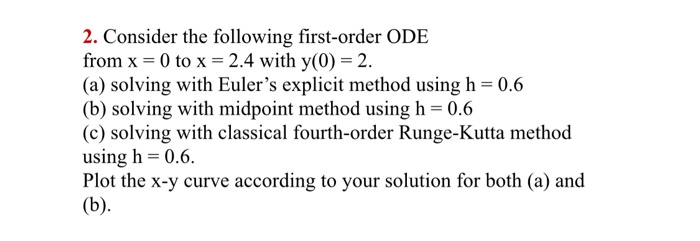2. Consider the following first-order ODE from x = 0 to x = 2.4 with y(0) = 2. (a) solving with Euler's explicit method using h=0.6 (b) solving with midpoint method using h= 0.6 (c) solving with classical fourth-order Runge-Kutta method using h = 0.6. Plot the x-y curve according to your solution for both (a) and (b).

• ### du Consider the following Ordinary Differential Equation (ODE): = 2.5 + + +0.5 210 - 2.y?...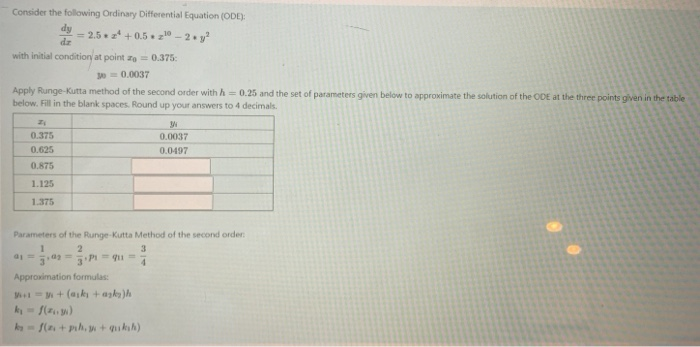du Consider the following Ordinary Differential Equation (ODE): = 2.5 + + +0.5 210 - 2.y? with initial condition at point o = 0.375: 300.0037 Apply Runge-Kutta method of the second order with h = 0.25 and the set of parameters given below to approximate the solution of the ODE at the three points given in the table below. Fill in the blank spaces. Round up your answers to 4 decimals. M 0.375 0.0037 0.625 0.0497 0.875 1.125 1.375 Parameters...

• ### Question 4 A mathematical model has been described by an engineer into the following differential equation: dy 0.5x0 dx...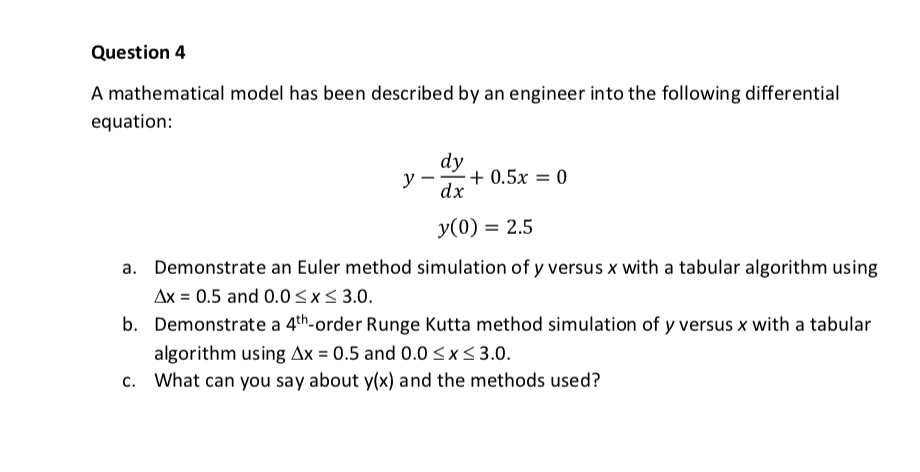Question 4 A mathematical model has been described by an engineer into the following differential equation: dy 0.5x0 dx y(0) 2.5 Demonstrate an Euler method simulation of y versus x with a tabular algorithm using a. 0.5 and 0.0 3.0. x Demonstrate a 4th-order Runge Kutta method simulation of y versus x with a tabular b. algorithm using дх-0.5 and 0.0 XS 3.0. What can you say about y(x) and the methods used? c. Question 4 A mathematical model has...

• ### The differential equation : dy/dx = 2x -3y , has the initial conditions that y = 2 , at x = 0 Obt...

The differential equation : dy/dx = 2x -3y , has the initial conditions that y = 2 , at x = 0 Obtain a numerical solution for the differential equation, correct to 6 decimal place , using , The Euler-Cauchy method The Runge-Kutta method in the range x = 0 (0.2) 1.0

• ### Help with these questions please. A mathematical model has been described by an engineer into the following differential equation: dy dx y(0) 2.5 Demonstrate an Euler method simulation of y versus x...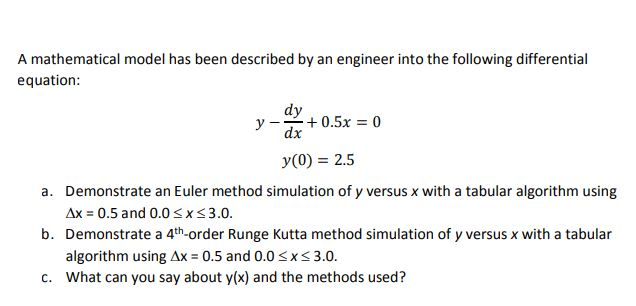Help with these questions please. A mathematical model has been described by an engineer into the following differential equation: dy dx y(0) 2.5 Demonstrate an Euler method simulation of y versus x with a tabular algorithm using Ax 0.5 and 0.0 X 3.0. Demonstrate a 4th-order Runge Kutta method simulation of y versus x with a tabular algorithm using What can you say about y(x) and the methods used? a. b. Ax 0.5 and 0.0 3.0 x c. A mathematical...

Free Homework App